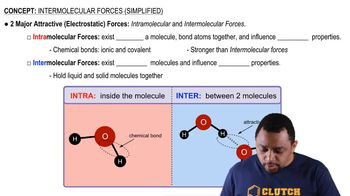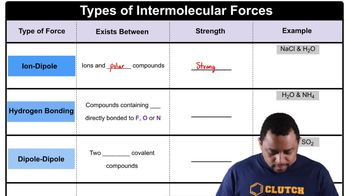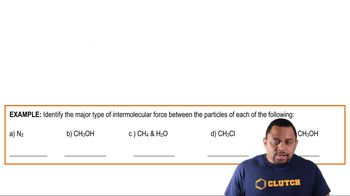Start typing, then use the up and down arrows to select an option from the list.# Intermolecular Forces

Pearson
126views
[ Music ] >> Intermolecular forces are the forces that exist between molecules. Most properties such as boiling point are directly proportional to the strength of the intermolecular forces of attraction between molecules. If we have a firm understanding of the intermolecular forces that exist in solutions, we can be able to predict properties such as a boiling point. Let's perform an experiment in which I have three liquids in front of me. The first is going to be hexane, the second heptane and the third octane. As I insert these three liquids into the sand bath, which one would you predict would boil first? As we can see, the hexane is boiling first, indicating it has the weakest intermolecular forces of attraction. Now which one of these two either the heptane or the octane will boil next? As we can see from the bubbles that are forming here in the middle tube the heptane will be the next liquid to boil. So now that we've seen each of these liquids boil in a particular order, we have to come up with a reason for why they boiled in the order that they did. Now if we look at these three non-polar molecules, hexane, heptane and octane, we see as we go from hexane to heptane to octane the molecular weight increases and what we observed in the boiling points here that was the liquid with the smaller molecular weight boiled first followed by the second largest molecular weight by the third largest molecular weight. We see that the dispersion forces that are in each of these three molecules increase as the molecular weight increases. This is why we see the hexane boil first, followed by the heptane, followed by the octane. Now let's take a look at acetic acid and 1-propanol. In this case, both of them have the same molecular weight. So if we came back up here to the sand bath and I took a solution here of 1-propanol and acetic acid and placed them in the sand bath, which one would you predict would boil first? So as we can see by the bubbles forming in this test tube over here, the 1-propanol is going to boil before the acetic acid. So now let's try to attempt to come up for a reason why these two particular liquids with the same molecular weight have a different boiling point. The dispersion forces are going to be the same because the molecular weight is the same. But these two liquids are going to be polar and both of them have hydrogen bonding but the difference here with the 1-propanol versus the acetic acid is that the acetic acid can form two hydrogen bonds between adjacent molecules while the 1-propanol can only form 1. So when we're looking at the general trends for intermolecular forces, these forces are going to be cumulative. So in addition to the dispersion forces we also have hydrogen bonding and the hydrogen bonding in the acetic acid is larger than it is in the 1-propanol and this is why we see the test tube over here on the left containing the 1-propanol will boil first.01:3904:0801:5901:5306:3601:59